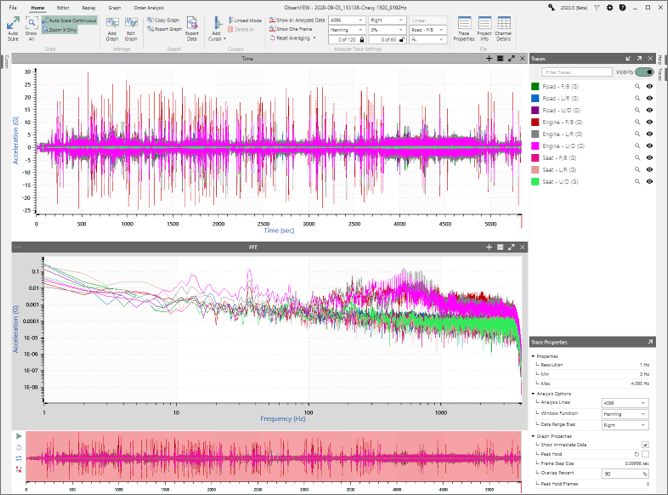# Analyze Data

March 31, 2021

ObserVIEW is used to analyze time waveform data; that is, separate data for evaluation. Common analysis methods in vibration testing include sampling, Fourier analysis, and wavelet analysis.

• Sampling: separating data into samples
• Fourier analysis: separating data into sinusoids
• Wavelet analysis: separating data into wavelets

Vibration data are collected in the time domain and can be analyzed in that domain. However, it is often advantageous to transform the data into a different domain for analysis. Patterns such as resonant frequencies that are not apparent in the time domain become readily apparent in other domains.

### The Fast Fourier Transform

In engineering, the frequency domain is the most common domain for analysis. For continuous data, the Fourier transform is used to project the time domain data into the frequency domain. The fast Fourier transform (FFT) uses sine or cosine waves of varying frequency, amplitude, and phase to do so.

In a complex signal, the FFT helps to determine the frequencies that are being excited and the amplitude at each frequency. Additionally, it highlights the changes at frequency and amplitude and the harmonic excitation in a frequency range.

To add an FFT graph in ObserVIEW, select Add Graph > FFT.FFT graph display in ObserVIEW.

The FFT settings include analysis lines, window function, and data bias range. The FFT assumes data to be continuous, and a window function is applied to minimize aliasing.

### Analyze Data

ObserVIEW includes several operations for analyzing data in the frequency domain.

#### Analyzer Traces

Analyze time waveform data in the frequency domain.

• Fast Fourier Transform (FFT): Transforms time-domain data into the frequency domain.
• Power Spectral Density (PSD): Displays the amount of power in a band of frequencies.
• Transfer Function: Identifies a resonance or antiresonance in a system.
• Coherence: Indicates how closely a pair of signals are statistically related.
• Cross Spectral Density: Displays the amount of power in the frequency band of a pair of signals that have been multiplied in the frequency domain.
• Transmissibility: Identifies a resonance or antiresonance in a system.

### Analysis Options

The analyzer trace settings are located under the Home ribbon menu and Trace Properties dialog.

#### ANALYSIS LINES

Determines the number of samples the software uses when analyzing the waveform. Along with the sample rate, analysis lines determine the frequency resolution of the analyzer.

#### ANALYSIS FRAMES AND AVERAGING DOF

The analysis frames value is the number of FFT windows the software uses to compute traces based on Welch estimators. The value can be entered manually entered and locked so the number of frames is not affected by zooming in and out on the time waveform graph.

The averaging degrees-of-freedom (DOF) value displays the statistical degrees of freedom associated with the PSD. After entering an averaging DOF value, the software automatically selects the analysis frames value to meet the target averaging DOF. Averaging DOF can also be locked.

#### WINDOW FUNCTIONS

Windowing provides a weighted selection of a portion of a time waveform for the purpose of FFT analysis. It is accomplished by multiplying the original time waveform by a window function of some selected width. The ObserVIEW Help file includes a comprehensive table of window functions for assistance with selection.